×#### Thank you for registering.

One of our academic counsellors will contact you within 1 working day.

Click to Chat

1800-1023-196

+91-120-4616500

CART 0

• 0

MY CART (5)

Use Coupon: CART20 and get 20% off on all online Study Material

ITEM
DETAILS
MRP
DISCOUNT
FINAL PRICE
Total Price: Rs.

There are no items in this cart.
Continue ShoppingAlgebra of Limit

Table of Content

Can a function have two distinct limits as x approaches 'a'?

We can use several methods for evaluating the limits. Some of the important ones include

Some important theorems

Some Important Results on Limits

Frequently Used Series Expansions

Limits at infinity and infinite limits

Related Resources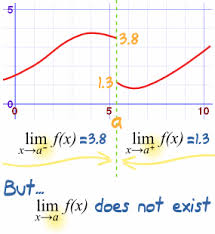We have already discussed the concept of limit of a function.

Let f be a function defined on an open interval containing a point ‘c’ (except possibly at c) and let us assume ‘L’ to be a real number.

Then, the function f is said to tend to a limit ‘L’ written as lim x→c f(x) = L if for each ∈ > 0, there exists a δ > 0 such that for all x satisfying 0 < |x – c| < δ, we have |f(x) – L| < ∈ or symbolically, we can define it as

∀ ∈ > 0, ∃ a δ > 0 such that 0 < |x – c| < δ ⇒ |f(x) – L| < ∈.

In this section, we shall mainly discuss the concept of algebra of limits and various important results

Suppose lim x→a f(x) = α and lim x→a g(x) = β then, if both α and β exist, then we can define the following rules:

lim x→a [f(x) + g(x)] = lim x→a f(x) + lim x→a g(x) = α + β

lim x→a [f(x) - g(x)] = lim x→a f(x) + lim x→a g(x) = α - β

lim x→a [f(x) . g(x)] = lim x→a f(x) + lim x→a g(x) = α . β

lim x→a fog(x) = f(lim x→a g(x)) = f(β), only if f is continuous at g(x) = β

lim x→a |f(x)| = |lim x→a f(x)| = |α|

lim x→a [f(x)]g(x) = lim x→a [f(x)]lim x→a g(x) = αβ

If lim x→a f(x) = + ∞ or -∞ then

If f(x) ≤ g(x) for every x in the neighborhood of a, then lim x→a f(x) ≤ lim x→a g(x).

For more on algebra of limits, view the following videoThe above rules are applicable only when both lim x→a f(x) and lim x→a g(x) exist separately.

We now prove two of the properties, the rest of the parts can be easily proved as the proof of all the rules will follow on similar lines:

(i) lim x→a (f(x) + g(x)) = lim x→a f(x) + lim x→a g(x)

To prove the above result we choose ∈, then by definition of limit, there exist δ1 and δ2 both greater than zero such thatLet δ = min (δ1, δ2) then by algebra, we have

|f(x) + g(x) – α – β| = |f(x) – α + g(x)  – β|

≤ |f(x) – α| + |g(x) - β|

< ∈ whenever 0 < |x - α| < δ

Hence lim x→a (f(x) + g(x)) = lim x→a f(x) + lim x→a g(x)

(ii) lim x→a (f(x).g(x)) = lim x→a f(x) . lim x→a g(x)

We have to prove that for ∀ ∈ > 0, there exists some δ > 0 such that |f(x)g(x) – αβ| < ∈ whenever 0 < |x-a| < δ

Now |f(x)g(x) - αβ| = |f(x)g(x) - αg(x) + αg(x) - αβ|

< |f(x)g(x) - αg(x)| + |αg(x) - αβ|

= ||f(x) - α| |g(x)| + |α| |g(x) - β|

From definition ∀ ∈ > 0, ∃ δ1, δ2 > 0 such that |f(x) – α | < ∈/3|β| whenever 0 < |x-a| < δ1  where β is the upper bound of g(x) in the neighborhood of x - a

and |g(x) – β| < ∈/2|α| whenever 0 < |x-α| < δ2

So, we have

Now |f(x)g(x) - αβ| = ||f(x) - α| |g(x)| + |α| |g(x) - β|

< ∈ /3|β|. 3|β|/ 2 + |α| .∈ /2|α|

= ∈

So, |f(x)g(x) - αβ| < ∈ and hence lim x→a (f(x).g(x)) = lim x→a f(x) . lim x→a g(x).

Illustration:

let f: R → R be such that f(1) = 3 and f’(1) = 6. The find the value of lim x→0 [f(1 + x)/ f(1)]1/x. (IITJEE 2002)

Solution:

Let y = [f(1 + x)/ f(1)]1/x

So, log y = 1/x [log f(1+x) – log f(1)]

So, lim x→0 log y = lim x→0 [1/f(1 + x) . f’(1 + x)]

= f’(1)/f(1)

= 6/3

log (lim x→0 y) = 2

lim x→0 y = e2Can a function have two distinct limits as x approaches 'a'?

If lim x→a f(x) exists then it is unique i.e. there cannot be two distinct numbers L1 and L2 such that when x → a the function tends to both L1 and L2. Hence if lim x→a f(x) = L1 and lim x→a f(x) = L2, then L1 = L2.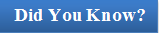If lim x→a f(x).g(x) exists then we can have the following cases:

Both the limits i.e. lim x→a f(x) and lim x→a g(x) exist.

lim x→a f(x) exists while lim x→a g(x) does not exist.

Consider f(x) = x and g(x) = 1/x, then lim x →0 f(x) g(x) exists and is equal to 1.

Also, lim x →0 f(x) exists and is equal to 0 but lim x →0 g(x) does not exist.

Both the limits i.e. lim x→a f(x) and lim x→a g(x) do not exist but even then lim x→a f(x).g(x) exists.

Similarly, if lim x→a [f(x) + g(x)] exists then we can have the following cases:

If ?lim x→a f(x) exists then lim x→a g(x) must exist.

This can be understood this way:

Since, g = (f + g) – f

So, by the limit theorem, lim x→a g(x) = [lim x→a f(x) + lim x→a g(x)] - lim x→a f(x).

Both the limits i.e. lim x→a f(x) and lim x→a g(x) do not exist.

Consider lim x→1 [x] and lim x→1 g{x}, where [.], {.} represent the greatest integer and fractional part function respectively. Here, both the limits do not exist but lim x→1 [x] + {x} = lim x→1 x = 1 exists.?

We can use several methods for evaluating the limits. Some of the important ones include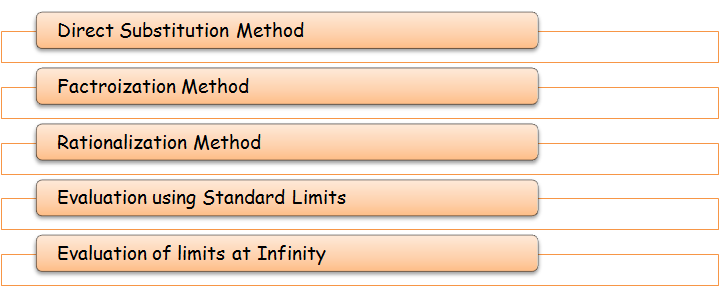1. Direct Substitution Method:

Consider the following limits (i) lim x→a f(x) and (ii) lim x→a φ(x)/Ψ(x).

If f(a) and φ(a)/Ψ(a) exist and are fixed real numbers and Ψ(a) ≠ 0, then we say that lim x→a f(x) = f(a) and lim x→a  φ(x)/Ψ(x) =  φ(a)/Ψ(a).

?2. Factorization Method:

Consider lim x→a f(x)/g(x). If by substituting x = a, f(x)/g(x) reduces to the form 0/0, then (x – a) is a factor of both f(x) and g(x). So, we first factorize f(x) and g(x) and then cancel out the common factor to evaluate the limit.

3. Rationalization Method:

This method is generally used in cases where either the numerator or the denominator or both involve expression consists of square roots and on substituting the value of x, the rational expression takes the form 0/0, ∞/∞.

4. Evaluation of Algebraic limits at infinity:

We know that lim x→∞ 1/x = 0 and lim x→∞ 1/x2 = 0.

Then lim x→∞ f(x) = lim y→0 f(1/y).Note:

When infinite limit is encountered i.e. '0' in the denominator as x→a, then either (x-a) gets cancelled from numerator and denominator both and if it is not possible then the limit's value is put as infinity. Such limits are called improper limits i.e. lim x→∞ 1/x2 = ∞.

Some important theorems

1. Let f < g in an open interval containing 'a' then lim x→a f(x) < lim x→a g(x).

2. Sandwich Theorem:

Let f, g, h be three continuous functions such that f < g < h in an open interval containing 'a' and suppose lim x→a f(x) = lim x→a h(x) = l. Then lim x→a g(x) also exists and is equal to 'l'.

Illustration:

As shown in figure given below, we have f ≤ g ≤ h.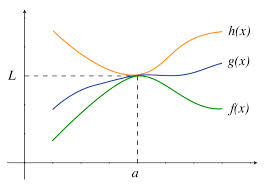In (x1, x2) as x approaches a, lim x→a f(x) = lim x→a  h(x) = lim x→a g(x) = 1.

3. If 0 ≤ f ≤ g in an open interval containing a and lim x→a g(x) = 0 then lim x→a f(x) exists and is equal to zero.

4. lim x→a f(x) = 0 if and only if  lim x→a f(x)Some Important Results on Limits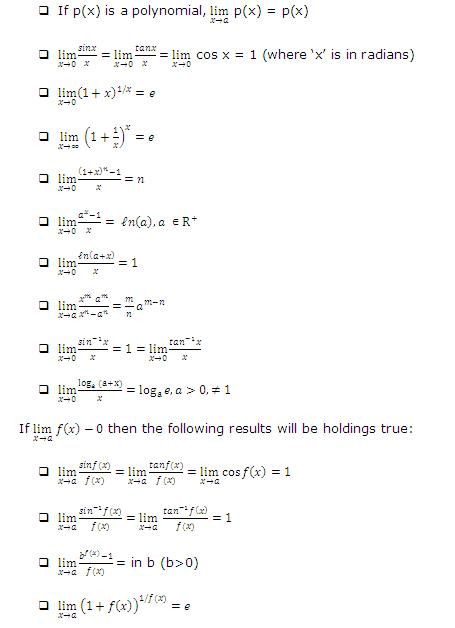Frequently Used Series Expansions

Following are some of the frequently used series expansions:Illustration:

Using the formulae evaluate the following

lim x→0 |x|/ x

Solution:

(1) The given function is

= lim x→0 (3 sin x - 4 sin3x)/x

= lim x→0 3(sin x /x) - lim x→0 4(sin x /x) (sin x)2

= 3 × 1 – 4 × 1 × 0 = 3.

(2) The given function is

?

= lim x→0 (((1 + (- x))1/-x)-1

= 1/eNote:

We know that

√(x2) = |x| = x ∀ x ≥ 0

= -x ∀ x < 0

lim x→0+ |x|/x = lim x→0+  x/x = lim x→0+ 1 = 1

Also, lim x→0- |x|/x = lim x→0- (-x)/x = lim x→0- -1 = -1

∴ lim x→0+ |x|/x ≠ lim x→0- |-x|/x

∴ lim x→0 |x|/x does not exist

Illustration:

Evaluate lim x→2 (x2 - 3x + 2)/(x2 - x – 2).

Solution:

Let f(x) = (x2 - 3x + 2)/(x2 - x – 2)

First, let us assume that x→ 2+

i.e. x = 2 + h, h → 0, h > 0

lim x→2+ f(x)

= lim h→0 f(2+h)

= lim h→0 ((2 + h)2 - 3(2 + h) + 2)/((2 + h)2 - (2 + h) - 2)

= lim h→0  h(1 + h)/h(3 + h) (since h ≠ 0, we cancel h)

= lim h→0 (1 + h)/(3 + h)

= (1 + 0)/(3 + 0) = 1/3

Now, consider the case when x → 2- i.e. x = 2 – h, h → 0, h > 0

lim x→2-  f(x) = lim h→0 f(2 – h)

= lim h→0  ((2 – h)2 - 3(2 – h) + 2)/((2 - h)2- (2 – h) – 2)

= limh→0 ((h2 - h)/(h2 - 3h))

= lim h→0 (h – 1)/(h – 3)

= (0 – 1)/(0 – 3) = 1/3

∴ lim x→2+ f(x) = lim x→2- f(x) = 1/3

Limits at infinity and infinite limits

When the term limits at infinity is used, it obviously means the evaluation of limit of functions as x→ ∞

For example lim x→∞ 1/x2 = 0

The term infinite limit means that when x tends to a particular value 'a'. Then the limit of the function tends to infinity i.e.lim x→2 f(x) = ∞

Evaluation of the limit at infinity in a problem is solved by changing the expression f(x) to g(1/x) form. Here, we have to find the value of the function f(x) as x→∞.

Illustration:

lim x→ ∞ ((3x – 1)(4x – 2))/((x – 8)(x - 1))

Solution:

lim x→ ∞ ((3x – 1)(4x – 2))/((x – 8)(x - 1))

lim x→ ∞ ((3 – 1/x)(4 – 2/x))/((1 – 8/x)(1 – 1/x))

Given expression has been changed from f(x) to g(1/x) form.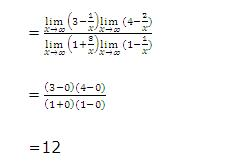_______________________________________________________________

Illustration:

For x ∈ R,

(IIT JEE 2000)

Solution:

= e-5Note:

When infinite limit is encountered i.e. '0' in the denominator as x→a, then either (x – a) get cancelled from numerator and denominator both and if it is not possible then the limit's value is put as infinity. Such limits are called improper limits i.e. limx→∞ 1/x2 = ∞.Q1. If lim x→a f(x).g(x) exists then

(a) both the limits lim x→a f(x) and lim x→a g(x) must exist.

(b) atleast one of them must exist.

(c) neither of them can exist.

(d) none of these

Q2. If lim x→a (f(x) + g(x)) exists then

(a) both the limits lim x→a f(x) and lim x→a g(x) must exist.

(b) if one exists then the other must exist

(c) limit of sum of two functions cannot exist

(d) none of these

Q3. lim x→0 |x|/x

(a) does not exist.

(b) exists.

(c) may or may not exist.

(d) none of these

Q4. lim x→a f(g(x)) = f(lim x→a (g(x)) = f(m)

(a) is always true

(b) true provided f is continuous at g(x) = m

(c) can never be true

(d) none of these

Q5. lim x→0 (1+x)1/x = ?

(a) 1

(b) 0

(c) e

(d) ∞Q1.

Q2.

Q3.

Q4.

Q5.

(d)

(b)

(a)

(b)

(c)

Related Resources

For getting an idea of the type of questions asked, refer the previous year papers.

You may also lke to refer L’ Hospital’s Rule.

To read more, Buy study materials of Limtis and Continuity comprising study notes, revision notes, video lectures, previous year solved questions etc. Also browse for more study materials on Mathematics here.### Course Features

• 731 Video Lectures
• Revision Notes
• Previous Year Papers
• Mind Map
• Study Planner
• NCERT Solutions
• Discussion Forum
• Test paper with Video Solution